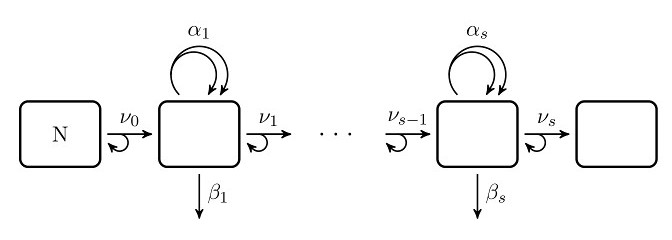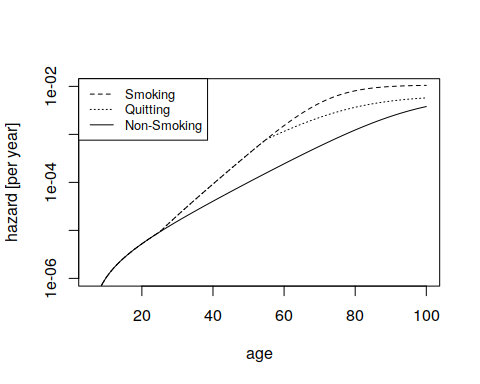Fitting Incidence Data with Multi-Stage Clonal Expansion Models using msce

Motivation

The Two-Stage Clonal Expansion Model was invented in the late 70's to explain cancer incidence curves by viewing carcinogenesis as a sequence of stochastic processes like mutations and proliferation of cells (Moolgavkar and Venzon 1979). Later on the model was successively extended to Multi-Stage Clonal Expansion (MSCE) Models (Little, Vineis, and Li 2008) and is regularly used to describe incidence data after time-dependend exposure to risk factors, especially in the field of radiation epidemiology (Rühm, Eidemüller, and Kaiser 2017).

Effects of risk factors on incidence (or mortality) data are fitted in MSCE models based on their presumed effect on disease devlopment. The model depends on so called biological parameters including e.g. mutation or proliferation rates. A risk factor is assumed to affect one or several of these biological parameters and the model relates this (time dependent) change in biological parameters to a change in the incidence risk. The resulting time-dependence of incidence risk may be perceived to be more realistic compared to fitting simple mathematical functions, and some insight between age- or time-dependence of risk and underlying processes may be gained. On the other hand, applying Multi-Stage Clonal Expansion Models for fitting of incidence data can be very intense regarding computing power, especially if the data set contains many strata (or individual person data) and biological parameters depend on age or time. To improve on this issue, the package msce was developed and takes advantage of the package RcppParallel to speed up calculations.

A glimpse on Multi-Stage Clonal Expansion Models

MSCE models are a sort of Markov process with countably infinite state space. Here, we do not present any mathematical details (Tan 1991) but give only one possible though simplified interpretation of its use in cancer epidemiology. Let's assume there is some mass $$N$$ of potentially cancerogenous cells (e.g. stem cells of a certain organ). Each of these cells has the same probability to acquire a certain transformation (mutation). This happens with rate $$\nu_0$$. Other transformations may follow with rates $$\nu_1$$ ... $$\nu_{s}$$. In the model, each transformation corresponds to the transition into a higher stage and the last stage corresponds to malignant cells. Here, we assume transformation to occur during cell division, i.e. the number of cells in the lower stage is not affected by a transition into a higher stage. With each stage the cell may have gained a proliferative advantage compared to the neighboring tissue thus expanding clonally. Cell division rate in stage $$i$$ is denoted $$\alpha_i$$ and (stem) cell extinction rate (by e.g. differentiation) is denoted $$\beta_i$$. See the figure below for a schematic presentation of MSCE models.Schematic depiction of the MSCE model.

In the msce package, the logarithm of the survival function and the hazard are calculated. The survival function is the age-dependent probability for no malignant cell. As usual, the hazard is the derivative of the negative logarithm of the survival function. Therefore, the survival function is interpreted as the probability of not having (a specific) cancer, the hazard is the modeled incidence rate. In many applications a fixed lag-time is introduced to approximately take into account the time difference between occurrence of the first malignant cell and observation of (or even death from) cancer.

General cautions on fitting incidence data with Multi-Stage Clonal Expansion Models

In this package, there are essentially three different types of biological parameters: transition rates $$\nu_i$$, $$i=0,1,...,s$$, proliferation rates $$\alpha_i$$ and clonal expansion rates $$\gamma_i$$, $$i=1,...,s$$. Dynamics of the first transition is governed only by the product of the number of potentially cancerogenous cells $$N$$ with $$\nu_0$$. Therefore, the absolute rate of first transitions $$N\nu_0$$ is expected as function argument and not $$N$$ and $$\nu_0$$ separately. Moreover, clonal expansion rates $$\gamma_i$$ are defined by $\gamma_i = \alpha_i-\beta_i$ for $$\beta_i$$ being a death (or differentiation) rate. One might argue which of $$\alpha_i$$, $$\beta_i$$ and $$\gamma_i$$ most naturally describe the disease process. Homeostasis is regulated in the body and not an accidental result of the opposing effects of proliferation and death/differentiation. Deviation from homeostasis between the clone and surrounding tissue is described by $$\gamma_i$$. Therefore, $$\gamma_i$$ are expected as function arguments, together with $$\alpha_i$$ mainly for ease of internal calculations. But in any case, this choice of function arguments does not hinder the user to apply any combination of parameters as fit parameters.

Finally, it has to be noted that not all parameters are identifiable from incidence data. This is true already for the Two-Stage Clonal Expansion Model (Heidenreich, Luebeck, and Moolgavkar 1997) because the incidence curve is insensitive to a certain parameter combination. For models with more stages, best estimates can typically not be calculated for several parameters. Therefore, at least some parameters or combinations thereof need to be fixed in the fitting procedure. It is therefore necessary to have an idea about reasonable values of the parameters. Moreover, parameters may be correlated, and fits with different models may yield similar goodness-of-fit. Therefore knowledge on the modeled processes should be taken into account and results of fitting need to be interpreted cautiously.

A fitting approach

For this example, a hypothetical lung cancer data set is provided.

library(msce)
data("lungCancerSmoking")

The data set is organized in strata. Each stratum is defined by a range in risk factors and presents one row in the data set. To keep our example simple, we assume only age and smoking cigarettes to be relevant risk factors for lung cancer incidence. Therefore, next to the variables age, there are ageStart and ageQuit for the ages of smoking start and cessation, cigsPerDay for smoking intensity, and pyr and cases for the corresponding number of person years followed up and the number of lung cancer cases that occurred during these person years. For lung cancer, we keep with the simplest, i.e. the Two-Stage Clonal Expansion model. It corresponds to $$s=1$$ in the above figure. A specific function tsce is provided for the Two-Stage Clonal Expansion model based on exact analytical formulas.

But before, there is some preparatory work to do. For lung cancer we may assume a lag-time of 5 years. This is equivalent to the assumption that it takes 5 years for a malignant cell to grow to an observed cancer and means that observation of cancer is not affected by the smoking history within the last five years.

lungCancerSmoking$laggedAge <- lungCancerSmoking$age-5

Define binary variables to classify each stratum to belong to non-smokers, former, or current smokers.

#consider smoking for less than one year as non-smoker
lungCancerSmoking$nonSmoke <- (lungCancerSmoking$ageStart==lungCancerSmoking$ageQuit) | (lungCancerSmoking$ageStart >= lungCancerSmoking$laggedAge) lungCancerSmoking$exSmoke <- (lungCancerSmoking$ageQuit < lungCancerSmoking$laggedAge) &
!lungCancerSmoking$nonSmoke lungCancerSmoking$smoke <- !lungCancerSmoking$nonSmoke & !lungCancerSmoking$exSmoke

Next define a matrix of time intervals during which biological parameters are assumed to be constant and an indicator function for the time intervals smoked. (Only cigarette smoking is assumed to alter biological parameters.)

# t: nonSmoke: 0        0        laggedAge
#    exSmoke:  ageStart ageQuit  laggedAge
#    smoke:    0        ageStart laggedAge
t<-matrix(0,nrow=NROW(lungCancerSmoking),ncol=3)
t[,3] <- lungCancerSmoking$laggedAge t[lungCancerSmoking$smoke,2] <-lungCancerSmoking$ageStart[lungCancerSmoking$smoke]
t[lungCancerSmoking$exSmoke,2] <- lungCancerSmoking$ageQuit[lungCancerSmoking$exSmoke] t[lungCancerSmoking$exSmoke,1] <- lungCancerSmoking$ageStart[lungCancerSmoking$exSmoke]

# smInd: nonSmoke: 0 0 0
#        exSmoke:  0 1 0
#        smoke:    0 0 1
smInd <- matrix(0,nrow=NROW(lungCancerSmoking),ncol=3)
smInd[lungCancerSmoking$smoke,3] <- 1 smInd[lungCancerSmoking$exSmoke,2] <- 1

It is convenient to write a wrapper function to relate fit parameters to the function arguments of tsce.

wrap <-function(pars)
{
# assume alpha to be small and constant
alpha <- matrix(1,nrow=1,ncol=3)

Nnu0 <- matrix(exp(pars),nrow=1,ncol=3)
nu1 <- matrix(exp(pars),nrow=1,ncol=3)

# assume only gamma to depend on smoking
gamma <- matrix(pars,nrow=NROW(lungCancerSmoking),ncol=3) +
pars*smInd +
pars*smInd * (lungCancerSmoking$cigsPerDay>5) parList <- list(Nnu0=Nnu0, alpha=alpha,gamma=gamma,nu1=nu1) result <- tsce(t,parList) return (result$hazard)
}

Moreover, we need the objective function to minimize, in this case the normal Poisson log-likelihood

loglik <- function(pars)
{
return (-sum(dpois(lungCancerSmoking$cases, lungCancerSmoking$pyr*wrap(pars), log = TRUE)))
}

Finally everything is set up to fit the model against the data. Here we use nlminb to find the minimum of the log-likelihood

# use upper bounds to ensure gamma < alpha
minResult <- nlminb(start = c(-3,-14,0.1,0.05,0.0), objective = loglik, upper=c(0,0,0.3,0.3,0.3))
bestEstimates <- minResult\$par
bestEstimates
#>   -2.62085682 -12.98733657   0.08941883   0.02084876   0.03663581

Assuming validity of the data and appropriateness of the model and assumptions, this result could be interpreted such that smoking increases the growth advantage of initiated cells from 0.09 to 0.11 per year for smoking with less than 5 cigarettes per day and to 0.15 for more intense smoking. As a side remark it may be noted that similar best estimates would have been obtained with the more general msce_numerical instead of the exact tsce function, a bestEstimate of -2.62 -13.0 0.0898 0.0210 0.0368.

To conclude, let's illustrate the fit result with a plot on the incidence risk for life-long non-smokers, and on the effect of starting intense smoking at age 20, and quitting at age 50.